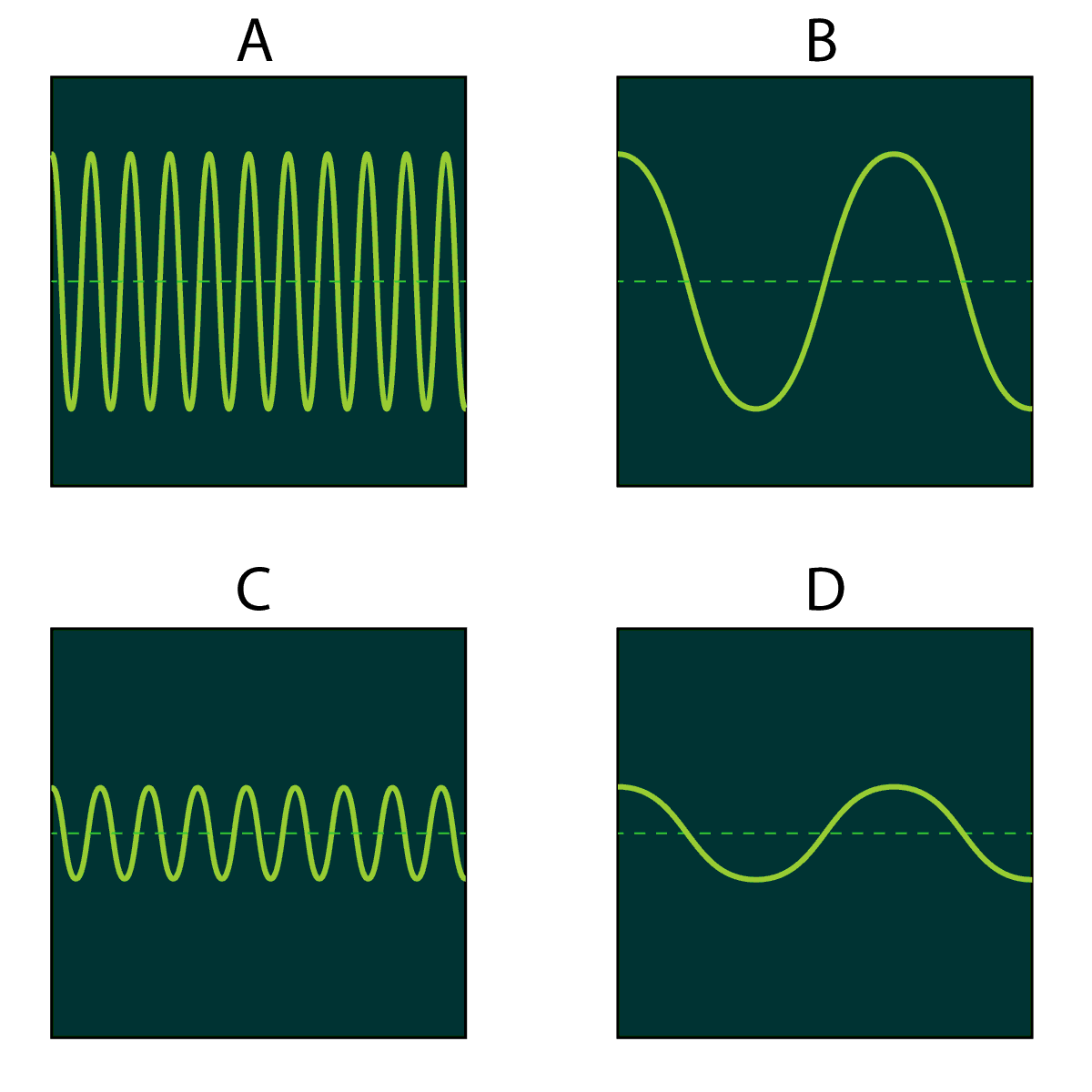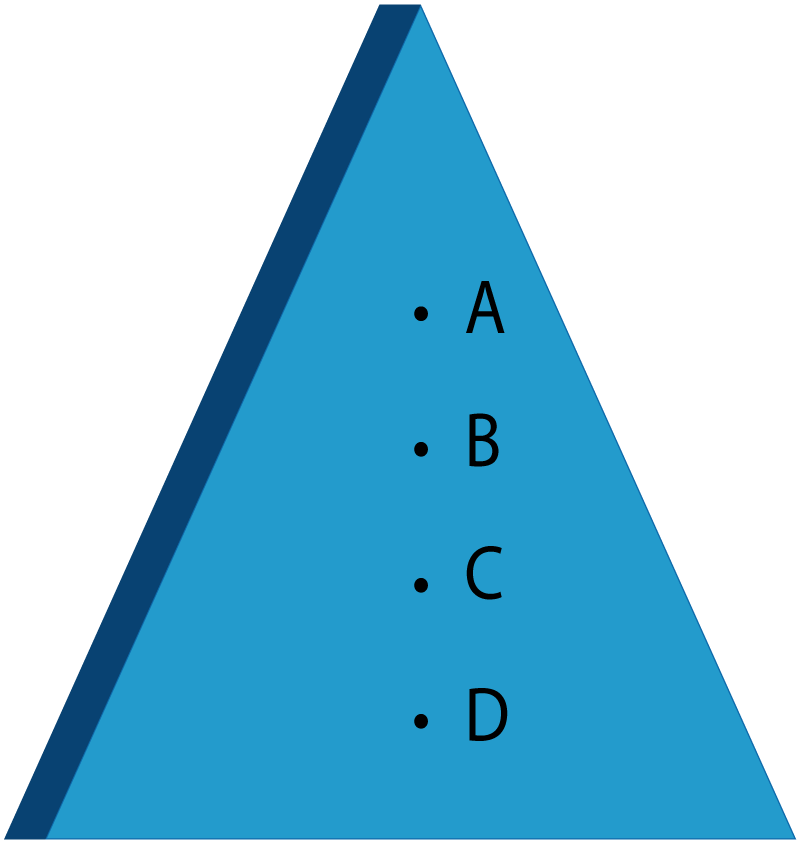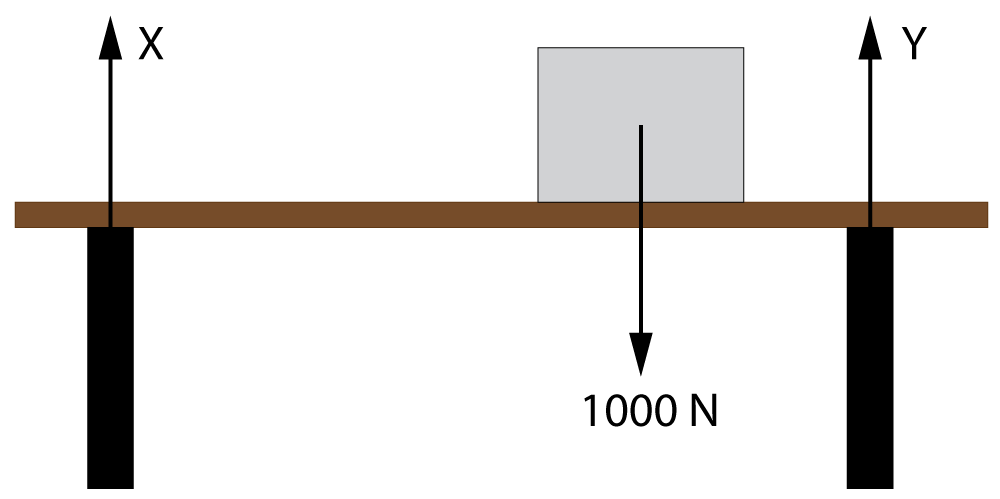NEED HELP? Edexcel iGCSE digital textbooks From the makers of Grade Gorilla. GGebooks.comSound, Forces, Energy Resources, Magnetism, Transformers. 1. What is the highest frequency humans can hear? A). 10 kHz B). 20 kHz C). 100 kHz D). 200 kHz ? A B C D 2. What is the lowest frequency humans can hear? A). 10 Hz B). 20 Hz C). 100 Hz D). 200 Hz ? A B C D 3. What do you call a device with a screen that can be used with a microphone to display a sound wave? A). Oscilloscope B). Stethoscope C). Sound sensor D). Signal generator ? A B C D 4&5. Here are 4 sound waves shown on one of these devices.Which of these diagrams shows... 4. A loud, high pitched sound? ? A B C D 5. A quiet high frequency sound? ? A B C D 6. A low frequency sound with a high amplitude? ? A B C D 7&8. In an experiment to measure the speed of sound, a student bangs together 2 wooden blocks. Another student listens out for the echo from the wall. The wall is 100 m away. The echo returns to the students after a delay of 0.6 seconds.7. Which of these 4 formulas should be used to calculate the speed of sound? A). speed = velocity x time B). speed = distance x time C). speed = velocity ÷ time D). speed = distance ÷ time ? A B C D 8. Using the data from this experiment, what is the speed of sound? A). 60 m/s B). 167 m/s C). 120 m/s D). 333 m/s ? A B C D 9. Which of the points on this metal block shows the position of the centre of gravity?? A B C D 10. The diagram below shows a light wooden beam supported at each end. A 1000 N weight rests on the beam closer to the right hand side of the beam.Which of the answers below gives realistic values for the size of the forces X and Y? A). X = 700 N, Y = 300 N. B). X = 300 N, Y = 700 N. C). X = 500 N, Y = 500 N. D). X = 1000 N, Y = 1000 N. ? A B C D